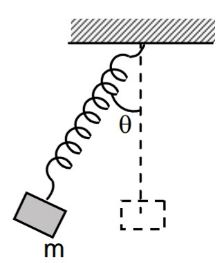# Spring-block system in an accelerated carA block of mass $m$ is suspended by an ideal spring of force constant $k$ from the ceiling of a car. The car is traveling at a constant speed along a circular path with a net acceleration $a$.

Due to the acceleration of the car the block and the spring system tilts by an angle $\theta$ with the vertical. In this position, the block is displaced slightly along the spring and released. What will be the time period of the small oscillations of the block?

×